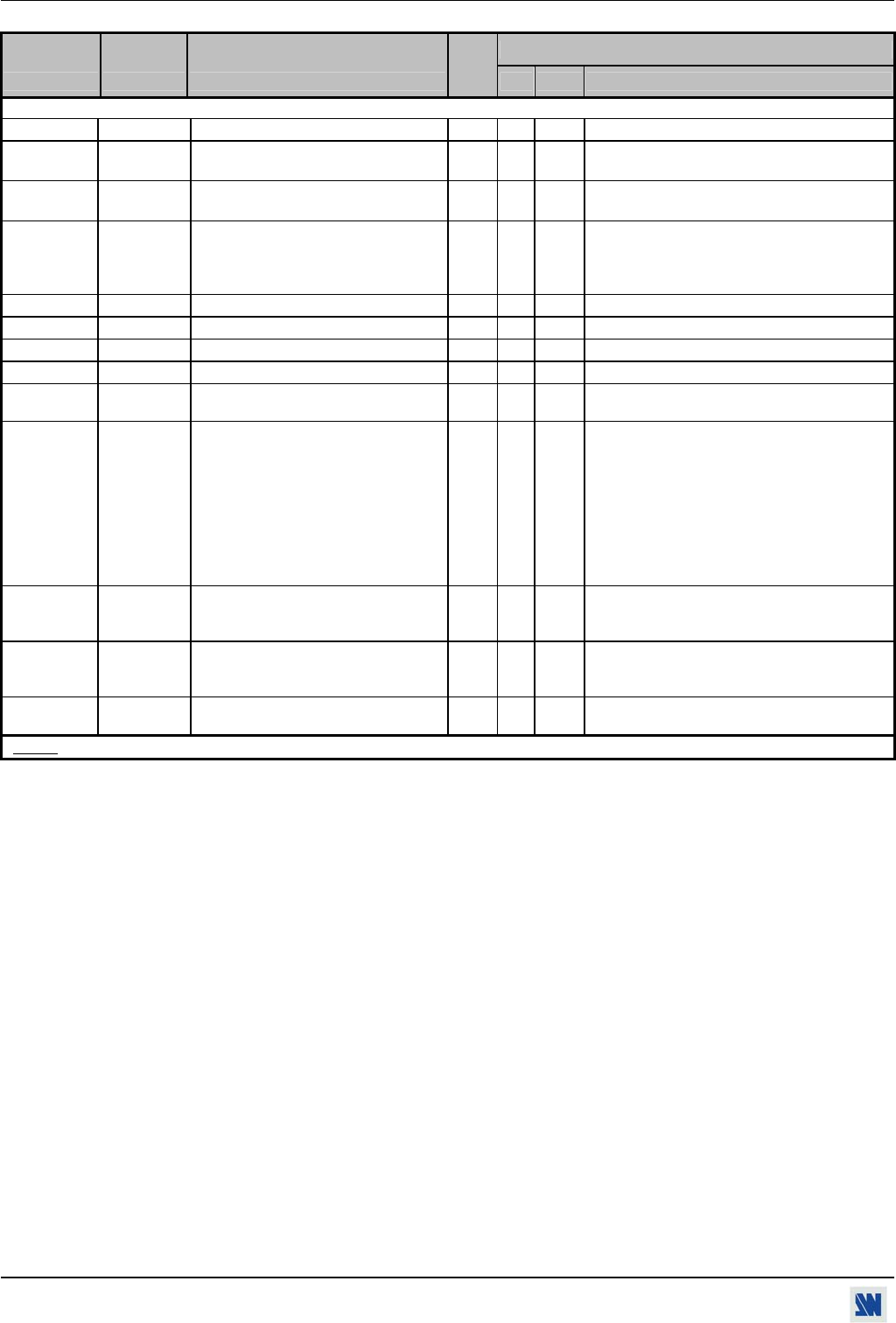APPENDIX A / ANNEXE A SCAN 1600 / DIGI SCAN 1600
PAGE 26
COMMAND RESPONSE COMMAND DESCRIPTION VALUE /VALEUR
COMMANDE RÉPONSE DESCRIPTION DE LA COMMANDE
TYPE
MIN MAX DESCRIPTION
STATUS COMMANDS / COMMANDES D’ETAT
U UNIT Measures unity in kHz.
Rd
0 65535
il ILD This command allows to calculate the
input line frequency in kHz
Rd
0 65535
Line frequency (in kHz) = (UNIT VALUE) ÷
(ILD VALUE).
id IFD This command allows to calculate the
input frame frequency in Hz.
Rd
0 65535 Frame frequency (in Hz) = (Line frequency in
Hz) ÷ (IFD VALUE).
ik IST Input sync type detection
Rd
0 3 0 = separate H & V
1 = Composite sync (TTL)
2 = SOG (Sync On Green)
3 = Composite sync (analog)
ip IPS Input sync detection.
Rd
0 1 0 = no sync detected 1 = sync detected
ih IHP Sign of the horizontal input sync.
Rd
0 1 0 = negative 1 = positive
iv IVP Sign of the vertical input sync.
Rd
0 1 0 = negative 1 = positive
ii IIN Interlaced signal detection.
Rd
0 1 0 = not interlaced 1 = interlaced
io IOO Status of the signal in comparison with
the input range frequency.
Rd
0 1 0 = in range 1 = out of range
if IFA Input format detection
Rd
0 8 0 = no signal
1 = "out of range" signal.
2 = VGA1 350l
3 = VGA2 400l
4 = VGA3 480l
5 = SVGA
6 = XGA
7 = SXGA
8 = UXGA
GA REFA Status of the synchronization mode
Rd
0 2 0 = internal
1 = genlock
2 = wrong signal.
GF REFF Status of the synchronized format
(genlock).
Rd
0 2 0 = no signal
1 = NTSC 59.94 Hz.
2 = PAL 50 Hz.
GT REFT Frame frequency of the synchronized
signal (genlock) (in hundredth of Hz)
Rd
0 65535
NOTE: Rd = Read only command / Commande de lecture. Rd/Wr = Read and write command / Commande de lecture et d'écriture.